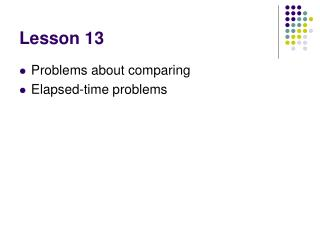DownloadDownload PresentationLesson 13

# Lesson 13

Download Presentation## Lesson 13

- - - - - - - - - - - - - - - - - - - - - - - - - - - E N D - - - - - - - - - - - - - - - - - - - - - - - - - - -
##### Presentation Transcript

1. Lesson 13 • Problems about comparing • Elapsed-time problems

2. Notes Mental Math Links Activity Proficiency Homework Practice

3. Mental Math • Count up and down by 3’s between 3 and 36. • 4 x 75 279 + 350 • 250 - 199 \$15 + \$7.75 • Double \$1.50 800/40 • 4 x 12, ÷ 6, x 8, - 4, ÷ 6, x 3, ÷ 2

4. Some Math Stories Are about Compare the Size of Two Groups Subtraction pattern Larger – smaller = difference L – S = D Example: There were 324 girls and 289 boys in the school. How many fewer boys than girls were there in the school? Use the 4-step process to solve the problem Step 1: subtraction pattern Step 2: larger – smaller = difference 324 – 289 = D Step 3: 324 girls – 289 boys = 35 sure boys Step 4: There were 35 fewer boys and girls in the school.

5. Elapsed-time Elapsed time is the length of time between two events Elapsed time problems are like comparison problems. They have a subtraction patterns. Later – earlier = difference L – E = D Example: How many years were there from 1492 to 1620? Step 1: Elapsed time problem (subtraction pattern) Step 2: 1620 – 1492 = D Step 3: 1620 - 1492 128 years Step 4: There were 128 years from 1492 to 1620.

6. http://www.math.com/school/subject1/lessons/S1U4L6GL.html http://www.kidsmath.com/e_int.html

7. A 1 ½ + ¾ = 2 ¼ 20 ¼ - 2 ¼ = 18 inches

8. A 1 ½ + ¾ = 2 ¼ 20 ¼ - 2 ¼ = 18 inches

9. Activity Tom followed the directions on the treasure map. Started at the big trees, he walked five steps north. Then he turned right and walked seven steps. He turned right again and walked nine steps. Then he turned left and walked three steps. Finally, he turned left and walked 4 steps. In what direction was he facing? How many steps was he from the big tree?

10. Lesson 13 Practice A-b Complete #’s 1-29

11. 24 17 of 24 :09 0 What is the perimeter of a square which 1 side is 25 mm? • 25 mm • 50 mm • 75 mm • 100 mm • None of the above

12. 24 18 of 24 :00 0 8 / 4 x 2 8 / (4 x 2) • < • > • = • None of the above

13. 24 18 of 24 :00 0 What is the next term in this sequence? 16, 24, 32, ___ • 38 • 40 • 64 • 96 • None of the above

14. 24 19 of 24 :09 0 What is the place value of the 1 in 12,453,000,000 • Ten trillion • Trillion • Ten billion • Billion • Ten thousand

15. 24 18 of 24 :08 0 What is the difference between the product of 6 and 4 and the sum of 6 and 4? • 8 • 12 • 14 • 24 • None of the above